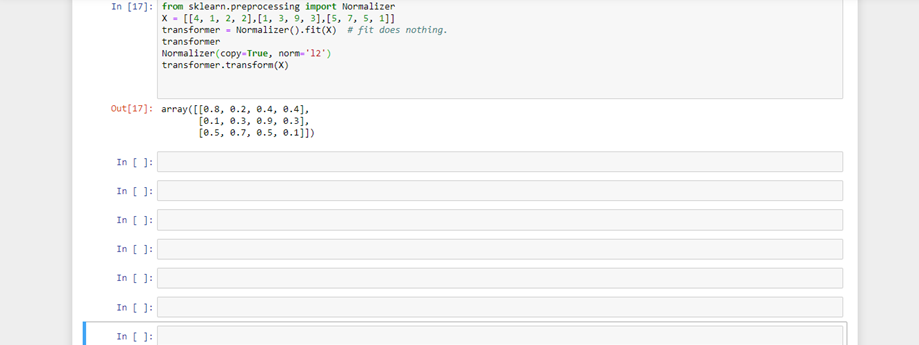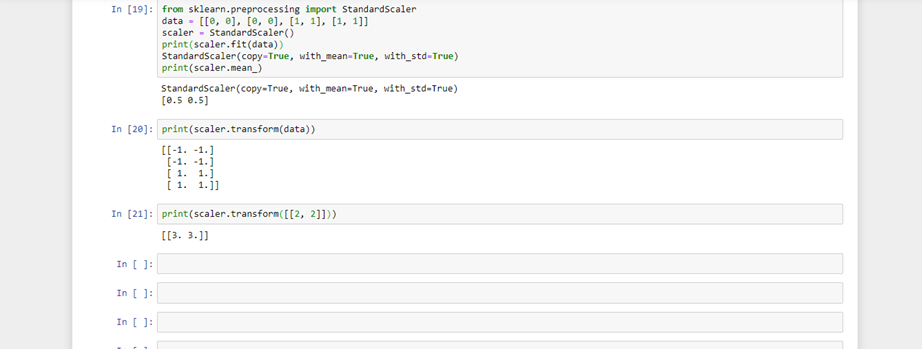+1 vote
2 views

What is the difference between standard scaler and normalizer in sklearn.preprocessing module? Don't both do the same thing? i.e remove mean and scale using deviation?

+1 vote
by (6.8k points)

Normalizer

Normalize samples individually to unit norm.

Each sample (i.e. each row of the info matrix) with a minimum of one non zero element is rescaled severally of alternative samples in order that its norm (l1 or l2) equals one.

This transformer is able to figure each with dense numpy arrays and scipy.sparse matrix (use CSR format if you would like to avoid the burden of a replica / conversion).

Scaling inputs to unit norms may be a common operation for text classification or cluster as an example. For instance the real number of two l2-normalized TF-IDF vectors is that the cosine similarity of the vectors and is that the base similarity metric for the Vector space Model normally used by the Information Retrieval community.

from sklearn.preprocessing import Normalizer

X = [[4, 1, 2, 2],[1, 3, 9, 3],[5, 7, 5, 1]]

transformer = Normalizer().fit(X)  # fit does nothing.

transformer

Normalizer(copy=True, norm='l2')

transformer.transform(X)StandardScalar

Standardize options by removing the mean and scaling to unit variance

The standard score of sample x is calculated as:

z = (x - u) / s

where u is that the mean of the training samples or zero if with_mean=False and s is that the variance of the training samples or one if with_std=False.

Standardization of a dataset could be a common demand for several machine learning estimators: they may behave badly if the individual options don't a lot of or less seem like standard normally distributed data (e.g. Gaussian with 0 mean and unit variance).

from sklearn.preprocessing import StandardScaler

data = [[0, 0], [0, 0], [1, 1], [1, 1]]

scaler = StandardScaler()

print(scaler.fit(data))

StandardScaler(copy=True, with_mean=True, with_std=True)

print(scaler.mean_)

print(scaler.transform(data))

print(scaler.transform([[2, 2]]))